# String Theory

## Notation

• Greek letters, e.g.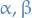, for variables or indices of variables
• Std. leteters, e.g.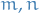, for indices of target space
•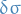denotes how the Lagrangian changes wrt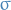## Just notes

• Gauge theories are used to obtain a "larger" space in which the symmetries (e.g. Lorentz invariance) becomes linear transforms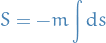where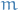is some constant (mass), and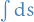is the arclength

• Nambu-Goto action: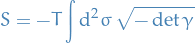where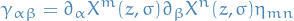with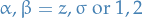, i.e.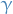a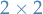matrix.

• We are pulling back the metric of the target space
• Symmetries
• Gauge symmetries:
• Reparametrizations \$δ σα = - ζα(z, σ)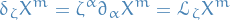• Std. parametrization: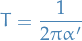where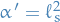is called the string length, and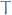is called tension (energy of unit of length of the string)

• Global symmetries:
•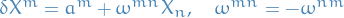(where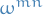are the Killing coefficients)
• Polyakov action: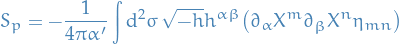where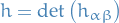and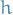is asymmetric metric.

1. No kinetic term implies the equations of motion are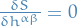, thus the symmetries become algebraic!
• Note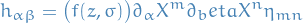for some function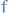, which we can solve for if we know the action, but in general it can be any, and so this is an example of a non-trivial Gauge symmetry

2. Symmetries of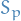1. Global: